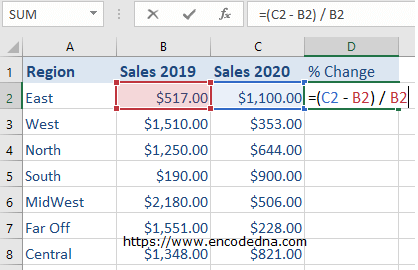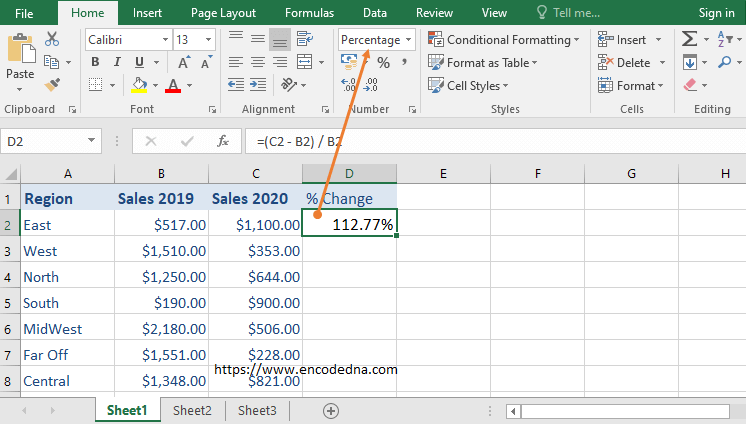﻿ Formula to Calculate Percentage Change in Excel

# Formula to Calculate Percentage Change in Excel

Let us assume, I have sales data in my Excel worksheet for two or more consecutive years (for certain products), which I collect from different regions. I want to know the percent change in sales figures during this period. The formula to get percent change is (new value – old value) / old value. I’ll show how you can use this formula in Excel.Syntax

(New Value – Old Value) / Old Value

See the image above. It show sales data for two years collected from different regions, followed by the % Change.

## The FormulaThe formula to calculate percent change is (New Value – Old Value) / Old Value. Remember, it uses BODMAS; therefore, it first calculates the values inside the braces (Subtracts) and then divides the result with the Old Value.

To apply this formula,

• Select cell D2
• Insert the formula by typing =(C2 – B2) / B2
• Press the Enter key

## Format Cell to Percentage

Once you have created the formula, it will show the value in \$ dollar (or General format) or whichever currency you choose. For example, considering the above data, (\$1,100 - \$517.00) / \$517.00 would result in \$1.13. This is default behavior; I mean that’s how Excel will calculate, since the 2nd and 3rd columns is of type Currency \$.

You’ll have to format the cells by changing the default (which is Currency) to Percentage. See the image.## Apply Formula to other Cells

Finally, set focus on cell D2, hover the mouse over the cell, you’ll see a little + sign, hold it and drag it down. It will write the formula in every row in D column.It will the % automatically when you format the cells to Percentage. Simple isn’t is.

You can apply the same formula if the data is in between the rows. You’ll just have to mention the cells, that you want to calculate in the formula.